# Proficiency Quiz Study Guide n Note The following

• Slides: 16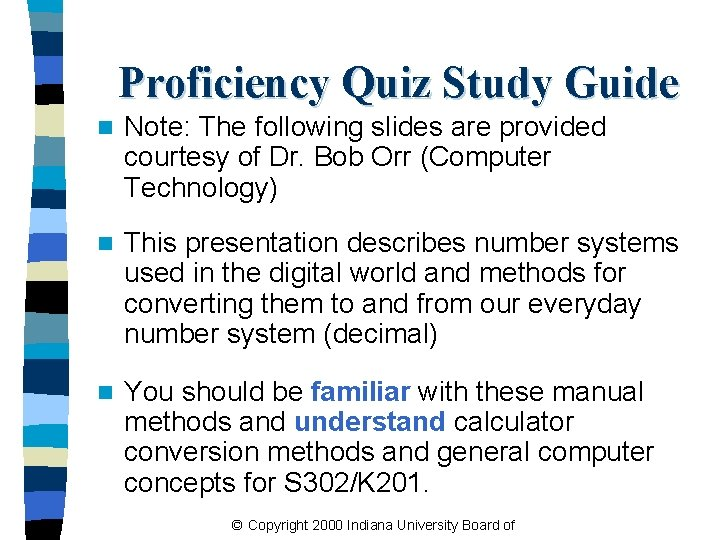Proficiency Quiz Study Guide n Note: The following slides are provided courtesy of Dr. Bob Orr (Computer Technology) n This presentation describes number systems used in the digital world and methods for converting them to and from our everyday number system (decimal) n You should be familiar with these manual methods and understand calculator conversion methods and general computer concepts for S 302/K 201. © Copyright 2000 Indiana University Board ofNumber Systems and Binary Arithmetic Quantitative Analysis II Professor Bob OrrIntroduction to Numbering Systems n We are all familiar with the decimal number system (Base 10). Some other number systems that we will work with are: – Binary Base 2 – Octal Base 8 – Hexadecimal Base 16 © Copyright 2000 Indiana University Board of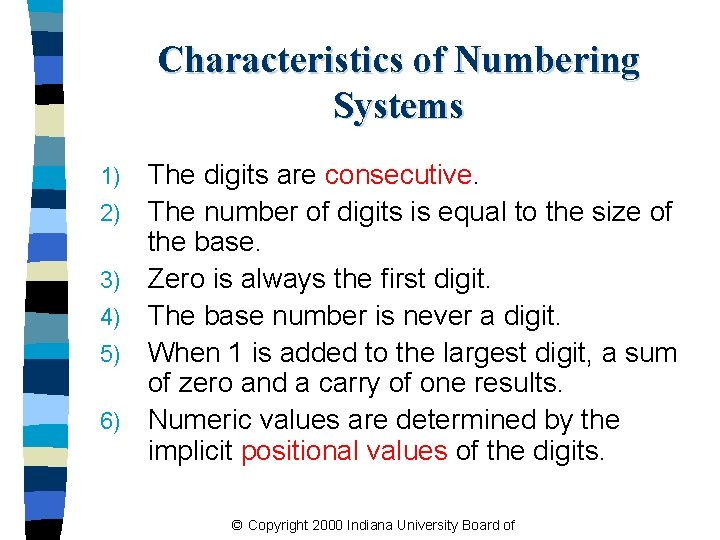Characteristics of Numbering Systems 1) 2) 3) 4) 5) 6) The digits are consecutive. The number of digits is equal to the size of the base. Zero is always the first digit. The base number is never a digit. When 1 is added to the largest digit, a sum of zero and a carry of one results. Numeric values are determined by the implicit positional values of the digits. © Copyright 2000 Indiana University Board of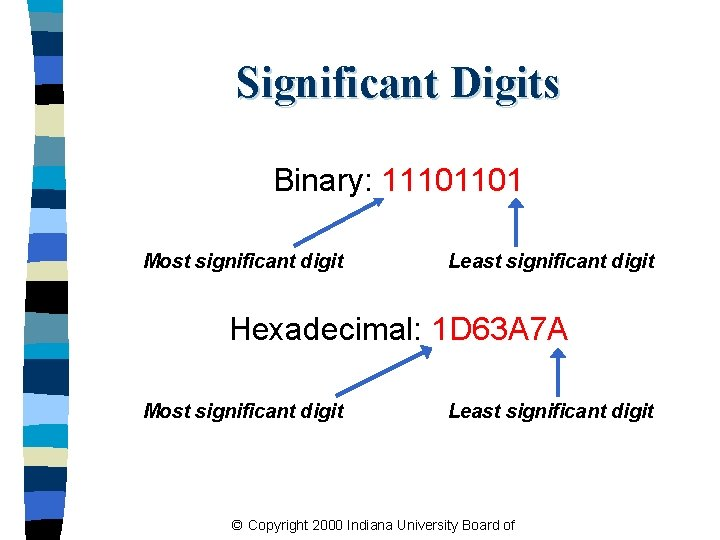Significant Digits Binary: 11101101 Most significant digit Least significant digit Hexadecimal: 1 D 63 A 7 A Most significant digit Least significant digit © Copyright 2000 Indiana University Board of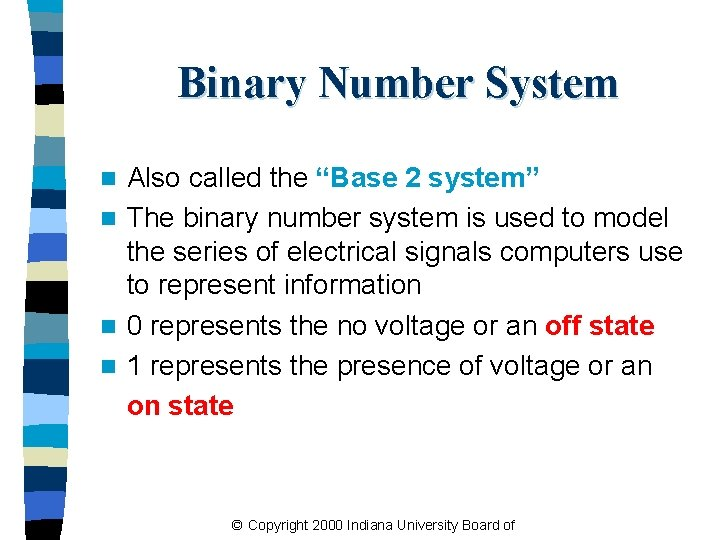Binary Number System Also called the “Base 2 system” n The binary number system is used to model the series of electrical signals computers use to represent information n 0 represents the no voltage or an off state n 1 represents the presence of voltage or an on state n © Copyright 2000 Indiana University Board ofBinary Numbering Scale Base 2 Number Base 10 Equivalent Power Positional Value 000 0 20 1 001 010 011 100 101 110 111 1 2 3 4 5 6 7 21 22 23 24 25 26 27 2 4 8 16 32 64 128 © Copyright 2000 Indiana University Board of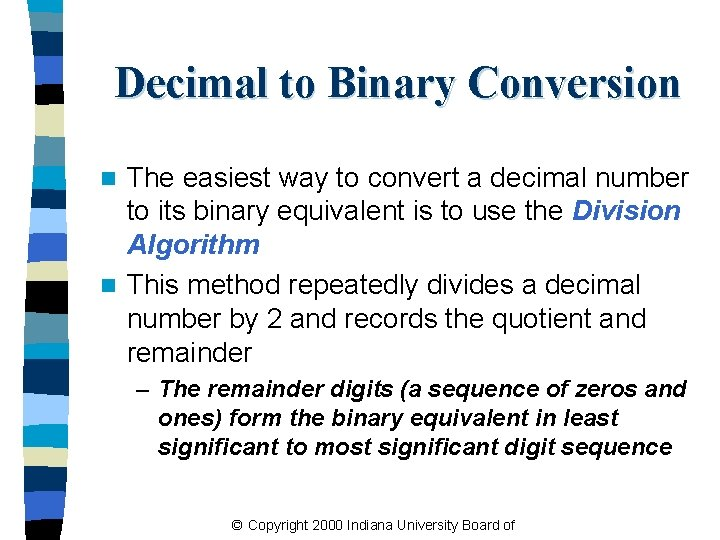Decimal to Binary Conversion The easiest way to convert a decimal number to its binary equivalent is to use the Division Algorithm n This method repeatedly divides a decimal number by 2 and records the quotient and remainder n – The remainder digits (a sequence of zeros and ones) form the binary equivalent in least significant to most significant digit sequence © Copyright 2000 Indiana University Board ofDivision Algorithm Convert 67 to its binary equivalent: 6710 = x 2 Step 1: 67 / 2 = 33 R 1 Divide 67 by 2. Record quotient in next row Step 2: 33 / 2 = 16 R 1 Step 3: 16 / 2 = 8 R 0 Step 4: 8 / 2 = 4 R 0 Step 5: 4 / 2 = 2 R 0 Step 6: 2 / 2 = 1 R 0 Step 7: 1 / 2 = 0 R 1 Again divide by 2; record quotient in next row Repeat again STOP when quotient equals 0 1 0 0 1 12 © Copyright 2000 Indiana University Board ofBinary to Decimal Conversion n The easiest method for converting a binary number to its decimal equivalent is to use the Multiplication Algorithm n Multiply the binary digits by increasing powers of two, starting from the right n Then, to find the decimal number equivalent, sum those products © Copyright 2000 Indiana University Board ofMultiplication Algorithm Convert (10101101)2 to its decimal equivalent: Binary 1 0 1 Positional Values 27 26 25 24 23 22 21 20 Products x x x x 128 + 32 + 8 + 4 + 1 17310 © Copyright 2000 Indiana University Board of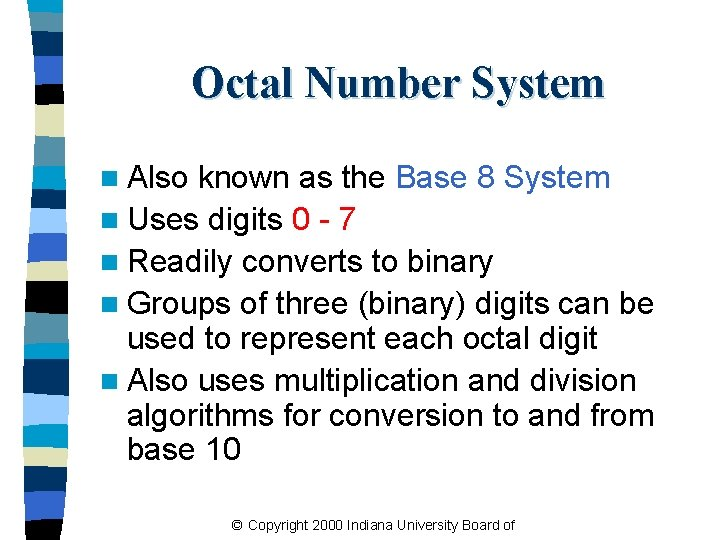Octal Number System n Also known as the Base 8 System n Uses digits 0 - 7 n Readily converts to binary n Groups of three (binary) digits can be used to represent each octal digit n Also uses multiplication and division algorithms for conversion to and from base 10 © Copyright 2000 Indiana University Board of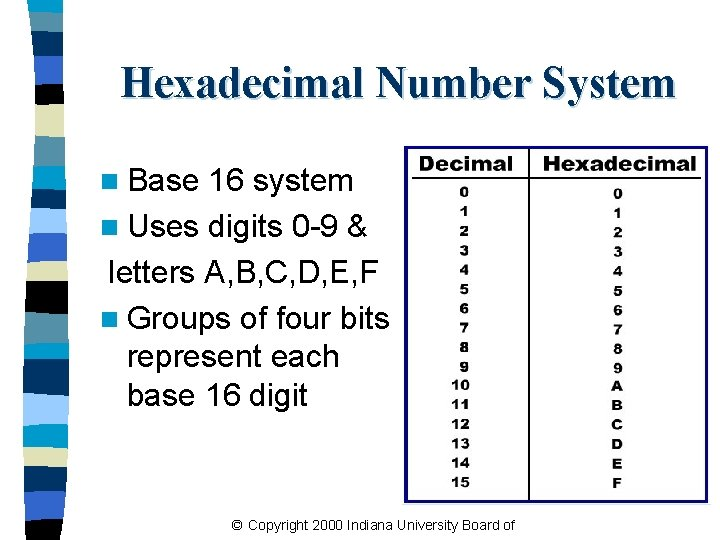Hexadecimal Number System n Base 16 system n Uses digits 0 -9 & letters A, B, C, D, E, F n Groups of four bits represent each base 16 digit © Copyright 2000 Indiana University Board of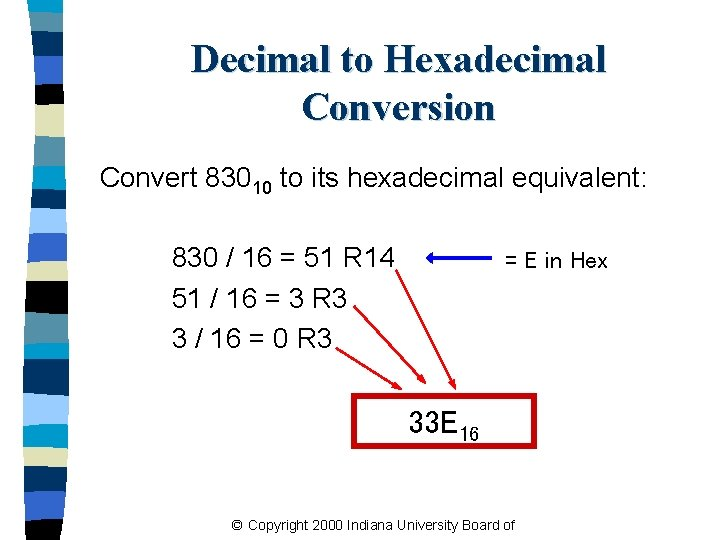Decimal to Hexadecimal Conversion Convert 83010 to its hexadecimal equivalent: 830 / 16 = 51 R 14 51 / 16 = 3 R 3 3 / 16 = 0 R 3 = E in Hex 33 E 16 © Copyright 2000 Indiana University Board ofHexadecimal to Decimal Conversion Convert 3 B 4 F 16 to its decimal equivalent: Hex Digits 3 Positional Values Products x B 4 x F x 163 162 161 160 12288 +2816 + 64 +15 15, 18310 © Copyright 2000 Indiana University Board of xBinary to Hexadecimal Conversion The easiest method for converting binary to hexadecimal is to use a substitution code n Each hex number converts to 4 binary digits n © Copyright 2000 Indiana University Board of